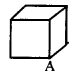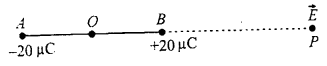# Bihar Board 12th Physics VVI Objective Questions Model Set 2 in English

Bihar Board 12th Physics Objective Questions and Answers

## Bihar Board 12th Physics VVI Objective Questions Model Set 2 in English

Question 1.
There are two types of electric charges positive charges and negative charges. The property which differentiates the two types of charges is
(a) field of charge
(b) amount of charge
(c) strength of charge
(d) polarity of charge
(d) polarity of charge

Question 2.
The magnetic flux linked with a coil of N turns of the area of cross-section A held with its plane parallel to the field B is
(a) $$\frac{N A B}{2}$$
(b) NAB
(c) $$\frac{N A B}{4}$$
(d) zero
(d) zeroQuestion 3.
Alternating voltage (V) is represented by the equation
(a) V(t) = Vm eωt
(b) V(t) = Vm sin ωt
(c) V(t) = Vm cos ωt
(d) V(t) = Vm tan ωt
where Vm is the peak voltage
(b) V(t) = Vm sin ωt

Question 4.
A 100 Ω resistor is connected to a 220 V, 50 Hz ac supply. The RMS value of current in the circuit is
(a) 1.56 A
(b) 1.56 mA
(c) 2.2 A
(d) 2.2 mA
(c) 2.2 A

Question 5.
Maxwell in his famous equations of electromagnetism introduced the concept of
(a) ac current
(b) displacement current
(c) impedance
(d) reactance
(b) displacement currentQuestion 6.
Displacement current goes through the gap between the plates of a capacitor when the charge on the capacitor
(a) is changing with time
(b) decrease
(c) does not change
(d) decreases to zero
(a) is changing with time

Question 7.
What can be the largest distance of an image of a real object from a convex mirror of radius of curvature is 20 cm?
(a) 10 cm
(b) 20 cm
(c) Infinity
(d) Zero
(a) 10 cm

Question 8.
A boy of height 1 m stands in front of a convex mirror. His distance from the mirror is equal to its focal length. The height of his image is
(a) 0.25 m
(b) 0.33 m
(c) 0.5 m
(d) 0.67 m
(c) 0.5 mQuestion 9.
The idea of secondary wavelets for the propagation of a wave was first given by
(a) Newton
(b) Huygens
(c) Maxwell
(d) Fresnel
(b) Huygens

Question 10.
Wavefront is the locus of all points, where the particles of the medium vibrate with the same
(a) phase
(b) amplitude
(c) frequency
(d) period
(a) phase

Question 11.
Who established that electric charge is quantised?
(a) J.J. Thomson
(b) William Crookes
(c) R.A Millikan
(d) Wilhelm Rontgen
(c) R.A Millikan

Question 12.
Cathode rays were discovered by
(a) Maxwell Clerk James
(b) Heinrich Hertz
(c) William Crookes
(d) J.J. Thomson
(c) William CrookesQuestion 13.
The first model of the atom in 1898 was proposed by
(a) Ernst Rutherford
(b) Albert Einstein
(c) J.J. Thomson
(d) Niels Bohr
(c) J.J. Thomson

Question 14.
In the Geiger-Marsden scattering experiment, the trajectory traced by an α-particle depends on
(a) number of collisions
(b) number of scattered α-particles
(c) impact parameter
(d) none of these
(c) impact parameter

Question 15.
The natural boron of atomic weight 10.81 is found to have two isotopes 10B and 11B. The ratio of abundance of isotopes of natural boron should be
(a) 11 : 10
(b) 81 : 19
(c) 10 : 11
(d) 19 : 81
(d) 19 : 81

Question 16.
The mass number of iron nucleus is 56, the nuclear density is
(a) 2.29 × 1016 kg m-3
(b) 2.29 × 1017 kg m-3
(c) 2.29 × 1018 kg m-3
(d) 2.29 × 1015 kg m-3
(b) 2.29 × 1017 kg m-3Question 17.
At absolute zero, Si acts as a
(a) metal
(b) Semiconductor
(c) insulator
(d) None of these
(c) insulator

Question 18.
In good conductors of electricity, the type of bonding that exist is
(a) Vander Walls
(b) covalent
(c) ionic
(d) metallic
(d) metallic

Question 19.
Who invented the world wide web?
(a) J.C.R. Licklider
(b) Tim Berners-Lee
(c) Alexander Graham Bell
(d) Samuel F.B. Morse
(b) Tim Berners-Lee

Question 20.
Essential elements of a communication system are
(c) transmitter and communication channel
(d) transmitter, communication channel and receiver
(d) transmitter, communication channel and receiverQuestion 21.
The total flux through the faces of the cube with a side of length a. if a charge q is placed at corner A of the cube is(a) $$\frac{\mathrm{q}}{8 \varepsilon_{0}}$$
(b) $$\frac{\mathrm{q}}{4 \varepsilon_{0}}$$
(c) $$\frac{\mathrm{q}}{2 \varepsilon_{0}}$$
(d) $$\frac{q}{\varepsilon_{0}}$$
(a) $$\frac{\mathrm{q}}{8 \varepsilon_{0}}$$

Question 22.
An object may acquire an excess or deficiency of charge by
(a) electric force
(b) heating
(c) shaking
(d) by rubbing
(d) by rubbing

Question 23.
The charge on an electron was calculated by
(b) J.J. Thomson
(c) Millikan
(d) Einstein
(d) Einstein

Question 24.
A method for charging a conductor without bringing a charged body in contact with it is called
(a) Magnetization
(b) Electrification
(c) Electrostatic induction
(d) Electromagnetic induction
(d) Electromagnetic inductionQuestion 25.
An object is charged when it has a charge imbalance, which means the
(a) the object contains no protons
(b) the object contains no electrons
(c) the object contains an equal number of electrons and protons
(d) the object contains an unequal number of electrons and protons
(d) the object contains an unequal number of electrons and protons

Question 26.
The number of electrons present in -1 C of charge is
(a) 6 × 1018
(b) 1.6 × 1019
(c) 6 × 1019
(d) 1.6 × 1018
(a) 6 × 1018

Question 27.
A cup contains 250 g of water. Find the total positive charges present in the cup of water.
(a) 1.34 × 1019 C
(b) 1.34 × 107 C
(c) 2.43 × 1019 C
(d) 2.43 × 107 C
(b) 1.34 × 107 C

Question 28.
Which of the following statement is not true?
(a) The electrostatic force is a conservative force.
(b) The potential at a point is the work done per unit charge in bringing a charge from infinity to that point.
(c) The electrostatic force is non-conservative.
(d) Potential is the product of charge and work.
(b) The potential at a point is the work done per unit charge in bringing a charge from infinity to that point.Question 29.
1 volt is equivalent to
(a) $$\frac{\text { newton }}{\text { second }}$$
(b) $$\frac{\text { newton }}{\text { coulomb }}$$
(c) $$\frac{\text { joule }}{\text { coulomb }}$$
(d) $$\frac{\text { joule }}{\text { second }}$$
(c) $$\frac{\text { joule }}{\text { coulomb }}$$

Question 30.
Two charges +20 µC and -20 µC are placed 10 mm apart. The electric field at point P, on the axis of the dipole 10 cm away from its centre O on the side of the positive charge is(a) 8.6 × 109 NC-1
(b) 4.1 × 106 NC-1
(c) 3.6 × 106 NC-1
(d) 4.6 × 105 NC-1
(c) 3.6 × 106 NC-1

Question 31.
1 ampere current is equivalent to
(a) 6.25 × 1018 electrons s-1
(b) 2.25 × 1018 electrons s-1
(c) 6.25 × 1014 electrons s-1
(d) 2.25 × 1014 electrons s-1
(a) 6.25 × 1018 electrons s-1Question 32.
Which one of the following is not correct about Lorentz Force?
(a) In presence of electric field $$\vec{E}(r)$$ and magnetic field $$\vec{B}(r)$$ the force on a moving electric charge is $$\vec{F}=q[\vec{E}(r)+\vec{v} \times \vec{B}(r)]$$
(b) The force, due to the magnetic field on a negative charge is opposite to that on a positive charge.
(c) The force due to the magnetic field becomes zero if velocity and magnetic field are parallel or antiparallel.
(d) For a static charge, the magnetic force is maximum.
(d) For a static charge, the magnetic force is maximum.

Question 33.
A strong magnetic field is applied on a stationary electron. Then the electron.
(a) moves in the direction of the field.
(b) remains stationary.
(c) moves perpendicular to the direction of the field.
(d) move opposite to the direction of the field.
(b) remains stationary.

Question 34.
The primary origin of magnetism lies in
(a) atomic current and intrinsic spin of electrons.
(b) polar and non-polar nature of molecules.
(c) Pauli exclusion principle.
(d) electronegative nature of materials.